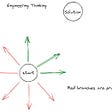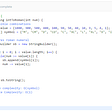# Java Review String Interview Exercises

`Input: address = "1.1.1.1"Output: "1[.]1[.]1[.]1"`
`Input: address = "255.100.50.0"Output: "255[.]100[.]50[.]0"`
• The given `address` is a valid IPv4 address.
`class Solution {    public String defangIPaddr(String address) {        StringBuilder st = new StringBuilder();        for(int i =0; i< address.length();i++){            if(address.charAt(i) == '.' ){                st.append("[.]");            }else{                st.append(address.charAt(i) );            }        }        return st.toString();    }}`

# Exercise #2

`Input: command = "G()(al)"Output: "Goal"Explanation: The Goal Parser interprets the command as follows:G -> G() -> o(al) -> alThe final concatenated result is "Goal".`
`Input: command = "G()()()()(al)"Output: "Gooooal"`
`Input: command = "(al)G(al)()()G"Output: "alGalooG"`
• `1 <= command.length <= 100`
• `command` consists of `"G"`, `"()"`, and/or `"(al)"` in some order.
`class Solution {    public String interpret(String command) {        int i =0;        StringBuilder st = new StringBuilder();        while(i <command.length() ){            if(command.charAt(i)=='G' ){   //G                st.append("G");                i++;            }else if("()".equals(command.substring(i,i+2)) ){ // ()                st.append("o");                i+=2;            }else if("(al)".equals(command.substring(i,i+4)) ){ // ()                st.append("al");                i+=4;            }        }        return st.toString();    }}`

# Exercise #3

`Input: allowed = "ab", words = ["ad","bd","aaab","baa","badab"]Output: 2Explanation: Strings "aaab" and "baa" are consistent since they only contain characters 'a' and 'b'.`
`Input: allowed = "abc", words = ["a","b","c","ab","ac","bc","abc"]Output: 7Explanation: All strings are consistent.`
`Input: allowed = "cad", words = ["cc","acd","b","ba","bac","bad","ac","d"]Output: 4Explanation: Strings "cc", "acd", "ac", and "d" are consistent.`
• `1 <= words.length <= 104`
• `1 <= allowed.length <= 26`
• `1 <= words[i].length <= 10`
• The characters in `allowed` are distinct.
• `words[i]` and `allowed` contain only lowercase English letters.
`class Solution {    public int countConsistentStrings(String allowed, String[] words) {        boolean[] isValid = new boolean;        int cnt=0;        //iterate through allowed chars marking isValid as true        fillAllowed(allowed,isValid);                //iter words to check no char is not allowed.        for(String word: words){            if(isWordAllowed(word,isValid) ) cnt++;        }        return cnt;    }    public boolean isWordAllowed(String word, boolean[] isValid){        for(char c: word.toCharArray() ){            if( !isValid[c-'a'] ) return false;        }        return true;    }    public void fillAllowed(String allowed, boolean[] isValid ){        for(char c: allowed.toCharArray() ){            isValid[c-'a'] = true;        }    }}`

# Exercise #4

`Input: s = "RLRRLLRLRL"Output: 4Explanation: s can be split into "RL", "RRLL", "RL", "RL", each substring contains same number of 'L' and 'R'.`
`Input: s = "RLLLLRRRLR"Output: 3Explanation: s can be split into "RL", "LLLRRR", "LR", each substring contains same number of 'L' and 'R'.`
`Input: s = "LLLLRRRR"Output: 1Explanation: s can be split into "LLLLRRRR".`
`Input: s = "RLRRRLLRLL"Output: 2Explanation: s can be split into "RL", "RRRLLRLL", since each substring contains an equal number of 'L' and 'R'`
• `1 <= s.length <= 1000`
• `s[i] = 'L' or 'R'`
`class Solution {    public int balancedStringSplit(String s) {        int i=0;        int rcount=0,            lcount=0;        int count =0;        while(i < s.length() ){            if(s.charAt(i)=='L' )lcount++;            if(s.charAt(i)=='R' )rcount++;            if( lcount==rcount ){                count++;                lcount=0;                rcount=0;            }            i++;        }        return count;    }}`

--

--

--

## More from Jesus PF

I am en electronics engineer, graduated from ITESM. Experienced working as functional validation and software development.

Love podcasts or audiobooks? Learn on the go with our new app.

## Some Useful Functions in Python## Creating a slack bot to for your changelogs — PART I: parsing## Kotlin: How var/val/const/lateinit Works Explained## Getting Started With Cross-Platform App Development In 2019## Why should Engineers focus on Problem Solving ?## Another Attempt To Overthrow VS Code: JetBrains Fleet## Today any turn follow so.## Jesus PF

I am en electronics engineer, graduated from ITESM. Experienced working as functional validation and software development.

## How To Create An Array Class in Java## “super” vs “this” in Java## Conversion Between Array and ArrayList in Java## 12. Integer to Roman | LeetCode | Medium | Java